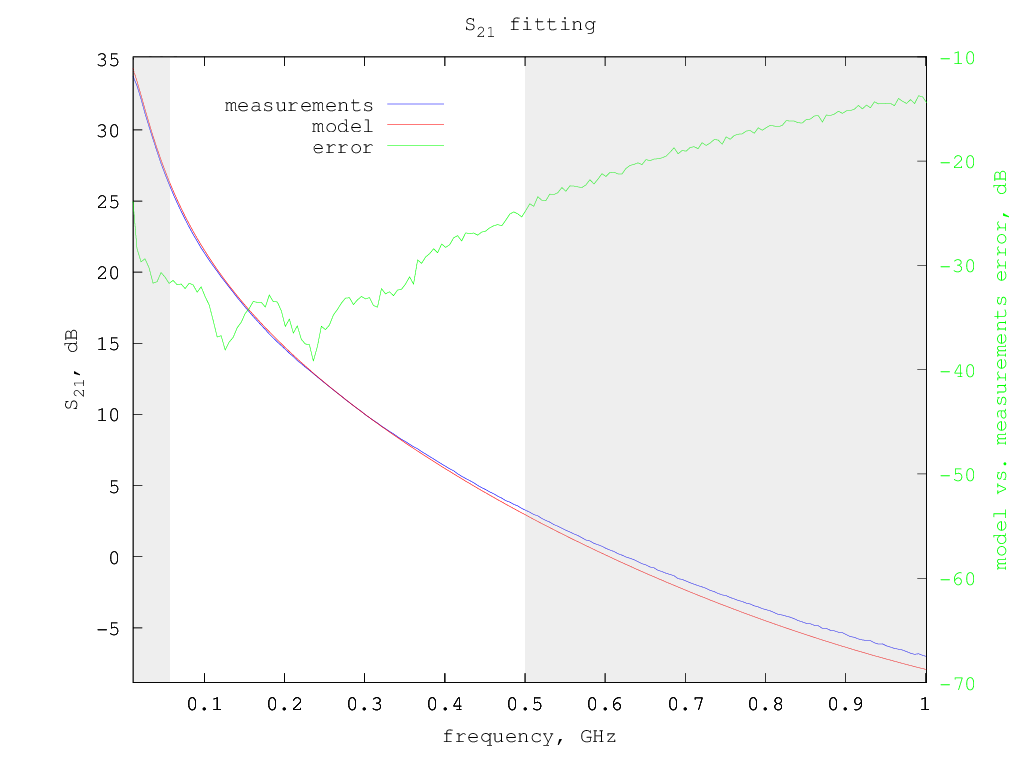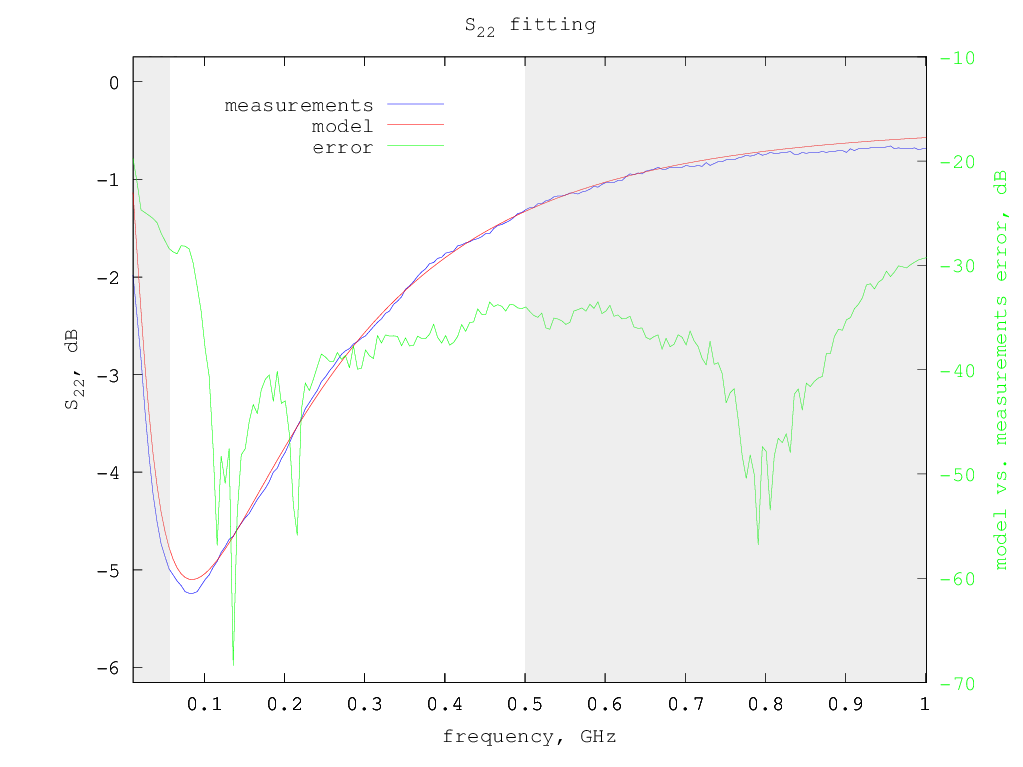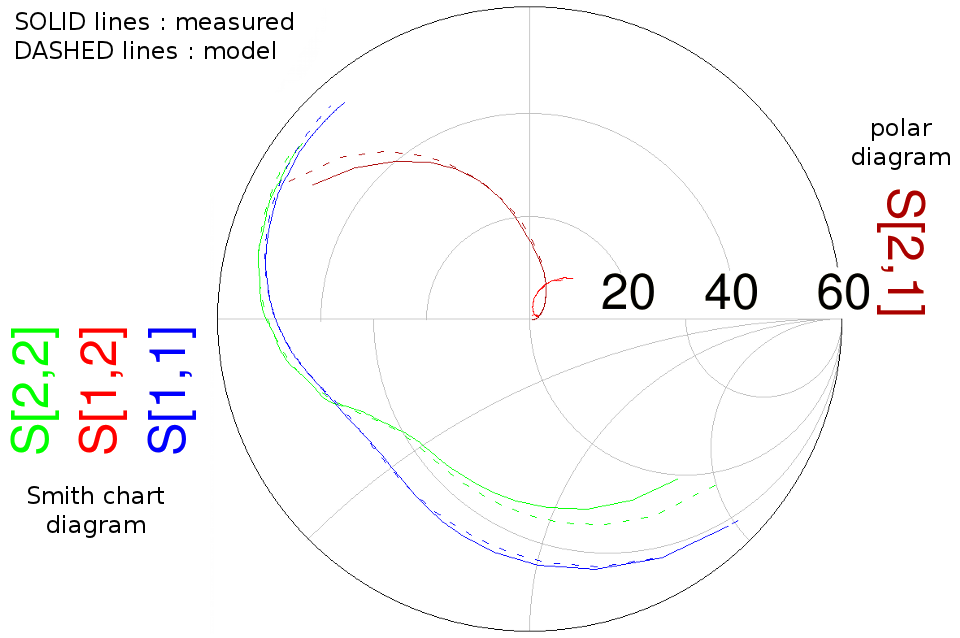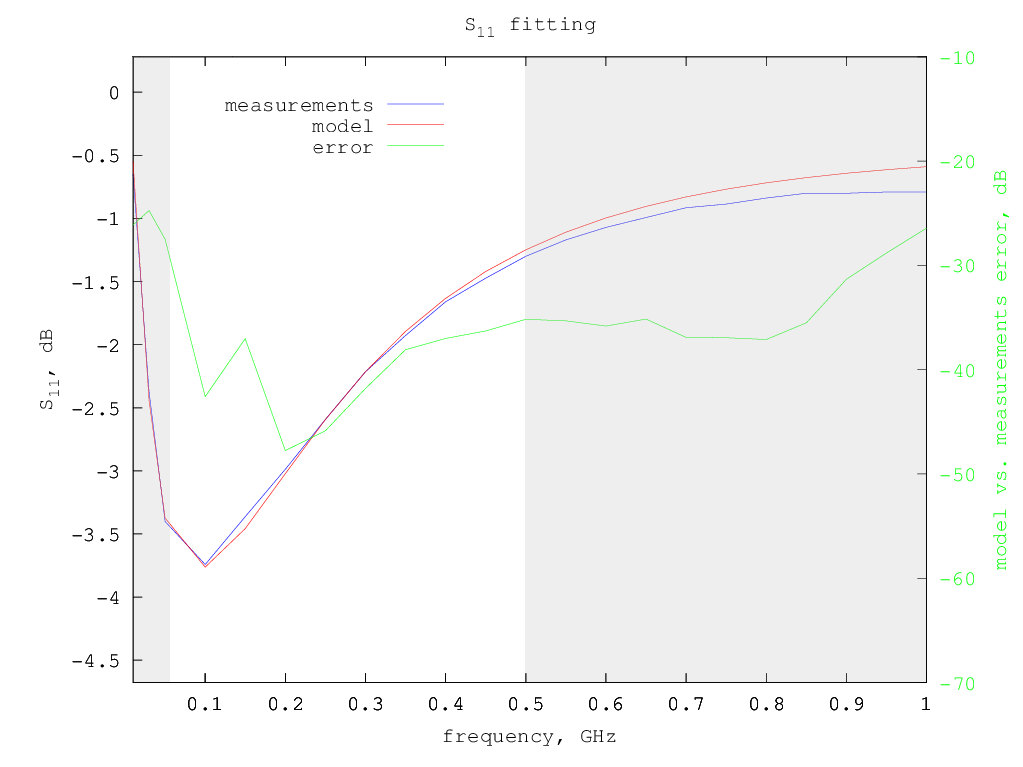# Mitsubishi RD16HHF1 LDMOS model

Under construction...

The Mitsubishi RD16HHF1 is a medium power LDMOS often used to build small amplifiers for amateur radio, either as a driver for a bigger device or standalone for lower output power.

# Model for Vdd=12.5 V and Idd= 500 mA

Typical S-parameters for this device can be found on the Mitsubishi web site, for a supply voltage of 12.5 V and a drain current of 500 mA.

Using the extraction method previously described, considering only the S-parameters from 50 MHz to 500 MHz, the following small-signal model parameters are determined:

```Rg = 0.987 ohm
Rd = 0.832 ohm
Rs = 0.111 ohm
Lg = 3.28 nH
Ld = 2.60 nH
Ls = 0.863 nH
Cgs = 43.5 pF
Cds = 31.9 pF
Cgd = 2.38 pF
gm = 0.639 S
gds = 0.525 mS
```
So the equivalent small-signal circuit for this LDMOS at 500 mA drain current is the following:Smith chart with measured vs. model S-parameters:S-parameters fitting details - fitted region is in white background:# Model for Vdd=12.5 V and Idd= 800 mA

The device datasheet contains also a table of S-parameters for Vdd=12.5 V and Idd=800 mA.

```Rg = 1.08 ohm
Rd = 0.808 ohm
Rs = 0.103 ohm
Lg = 3.30 nH
Ld = 2.64 nH
Ls = 0.872 nH
Cgs = 44.3 pF
Cds = 32.1 pF
Cgd = 2.44 pF
gm = 0.692 S
gds = 1.58 mS
```Smith chart with measured vs. model S-parameters:S-parameters fitting details - fitted region is in white background: